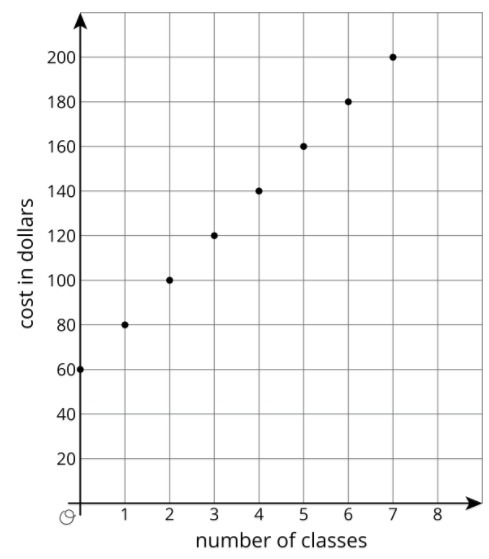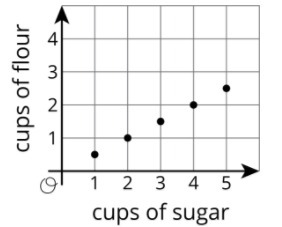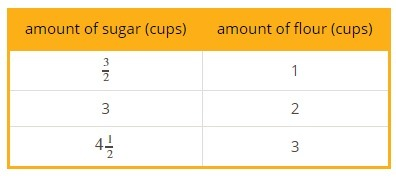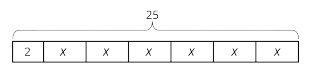PSABM5VT - Practice---Acc7.5 Lesson 5 More Linear Relationships (5.OA.B.3, 7.RP.A.2.a, 8.EE.B)

Explain what the slope and intercept mean in the situation.

A graph represents the perimeter, y, in units, for an equilateral triangle with side length x units. The slope of the line is 3 and the y-intercept is 0.

Explain what the slope and intercept mean in the situation.

The amount of money, y, in a cash box after x tickets are purchased for carnival games. The slope of the line isand the y-intercept is 8.

Explain what the slope and intercept mean in the situation.

The number of chapters read, y, after x days. The slope of the line isand the y-intercept is 2.

Explain what the slope and intercept mean in the situation.

The graph shows the cost in dollars, y, of a muffin delivery and the number of muffins, x, ordered. The slope of the line is 2 and the y-intercept is 3.

Part A)

Customers at the gym pay a membership fee to join and then a fee for each class they attend. Here is a graph that represents the situation.What does the slope of the line shown by the points mean in this situation?

Part B)

What does the vertical intercept mean in this situation?

Part A)

The graph shows the relationship between the number of cups of flour and the number of cups of sugar in Lin’s favorite brownie recipe.The table shows the amounts of flour and sugar needed for Noah’s favorite brownie recipe.Noah and Lin buy a 12-cup bag of sugar and divide it evenly to make their recipes. If they each use all their sugar, how much flour do they each need?

How much does Lin need?

Type your answer below as a number (example: 5, 3.1, 4 1/2, or 3/2):
Part B)

How much does Noah need?

Type your answer below as a number (example: 5, 3.1, 4 1/2, or 3/2):
Part C)

Noah and Lin buy a 10-cup bag of flour and divide it evenly to make their recipes. If they each use all their flour, how much sugar do they each need?

How much does Lin need?

Type your answer below as a number (example: 5, 3.1, 4 1/2, or 3/2):
Part D)

How much does Noah need?